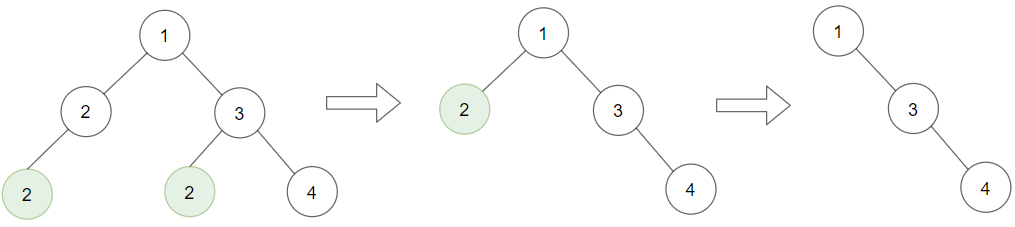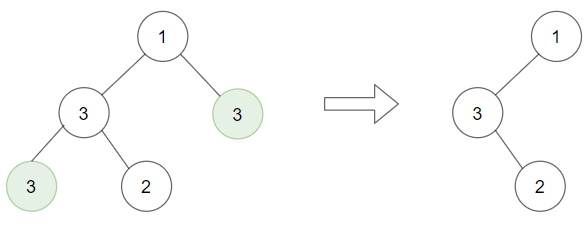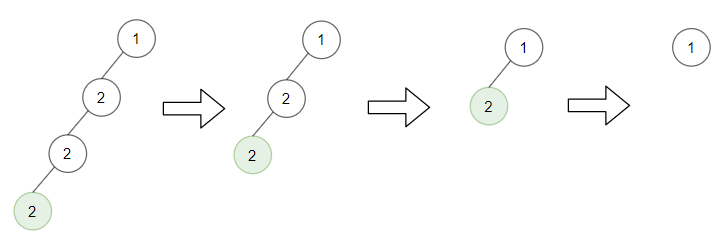# LeetCode: 1325. Delete Leaves With a Given Value

## 题目¶

Given a binary tree root and an integer target, delete all the leaf nodes with value target.

Note that once you delete a leaf node with value target, if it's parent node becomes a leaf node and has the value target, it should also be deleted (you need to continue doing that until you can't).

Example 1:```Input: root = [1,2,3,2,null,2,4], target = 2
Output: [1,null,3,null,4]
```

Explanation: Leaf nodes in green with value (target = 2) are removed (Picture in left). After removing, new nodes become leaf nodes with value (target = 2) (Picture in center).

Example 2:```Input: root = [1,3,3,3,2], target = 3
Output: [1,3,null,null,2]
```

Example 3:```Input: root = [1,2,null,2,null,2], target = 2
Output: 
```

Explanation: Leaf nodes in green with value (target = 2) are removed at each step.

Example 4:

```Input: root = [1,1,1], target = 1
Output: []
```

Example 5:

```Input: root = [1,2,3], target = 1
Output: [1,2,3]
```

Constraints:

• 1 <= target <= 1000
• The given binary tree will have between 1 and 3000 nodes.
• Each node's value is between [1, 1000].

## 解法¶

```# Definition for a binary tree node.
# class TreeNode(object):
#     def __init__(self, val=0, left=None, right=None):
#         self.val = val
#         self.left = left
#         self.right = right
class Solution(object):
def removeLeafNodes(self, root, target):
if root is None:
return

if root.left is None and root.right is None and root.val == target:
return

root.left = self.removeLeafNodes(root.left, target)
root.right = self.removeLeafNodes(root.right, target)

# 应对 note 提到的 case:
# Note that once you delete a leaf node with value target,
# if it's parent node becomes a leaf node and has the value target,
# it should also be deleted (you need to continue doing that until you can't).
if root.left is None and root.right is None and root.val == target:
return

return root
```